Function Repository Resource:

# NoiseSphere

Visualize the noise sphere of a set of random numbers

Contributed by: Jan Mangaldan
 ResourceFunction["NoiseSphere"][{r1,…,rn}] plots Pickover's noise sphere visualization of the numbers ri.

## Details

A noise sphere maps a sequence of uniformly-distributed random numbers to spherical coordinate triples. This is useful for visually detecting any unexpected correlations in the random number sequence.
The ri are taken to be in the range 0 to 1.
ResourceFunction["NoiseSphere"] takes the same options as Graphics3D.

## Examples

### Basic Examples (1)

Noise sphere for the default generator used by RandomReal:

 In:=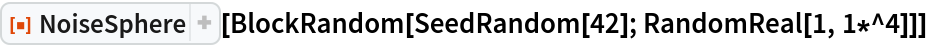Out=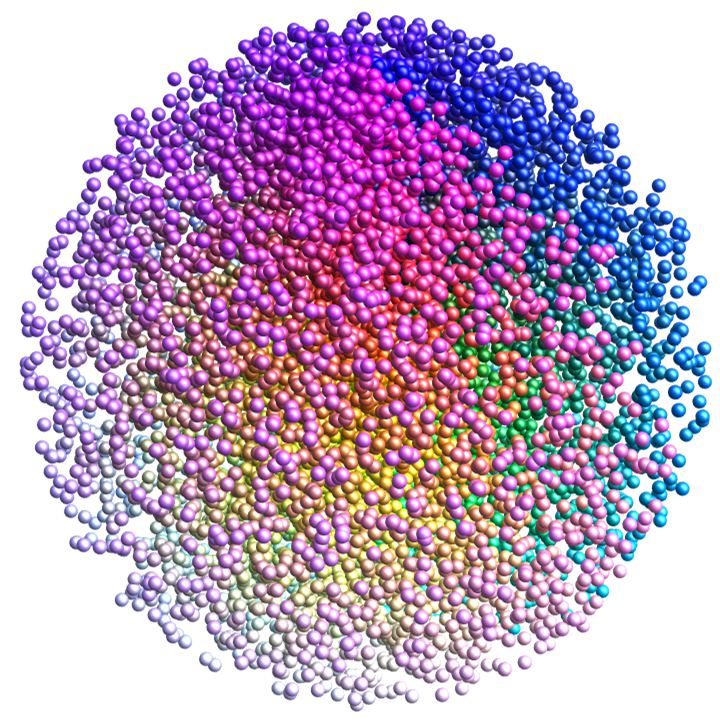### Scope (4)

Noise sphere of the IBM RANDU generator; the structured appearance suggests unwanted correlations:

 In:=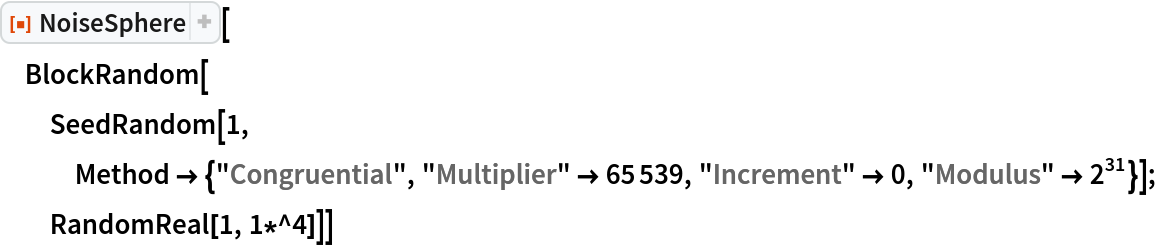Out=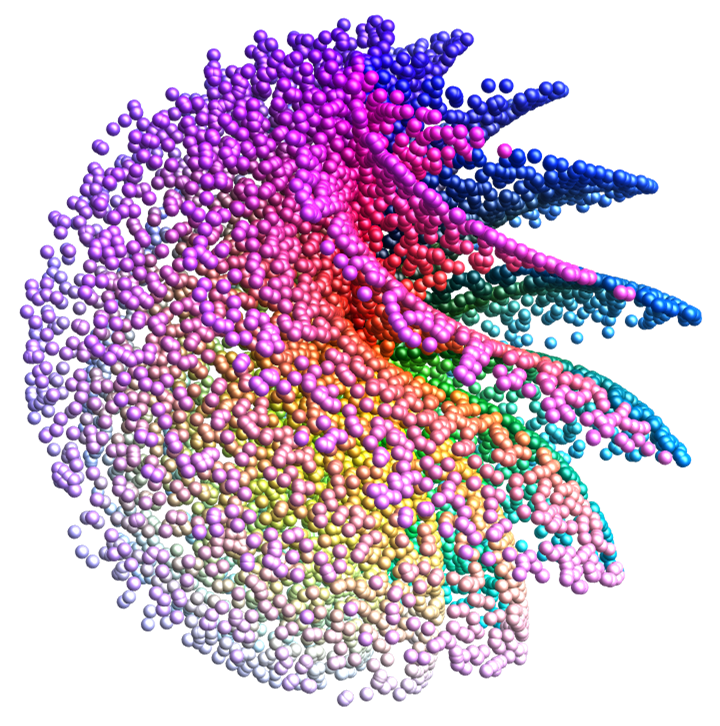Noise sphere of a linear congruential generator from L'Ecuyer:

 In:=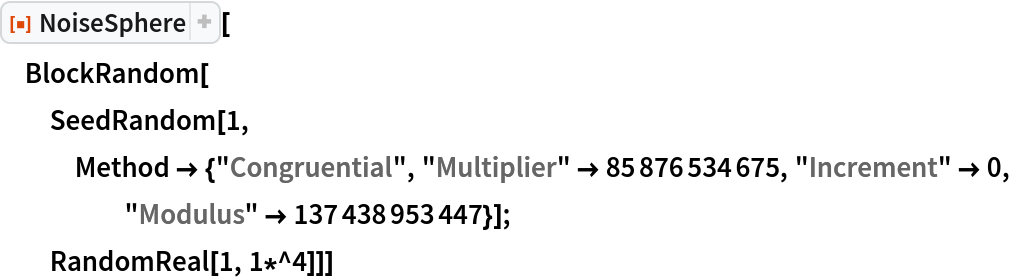Out=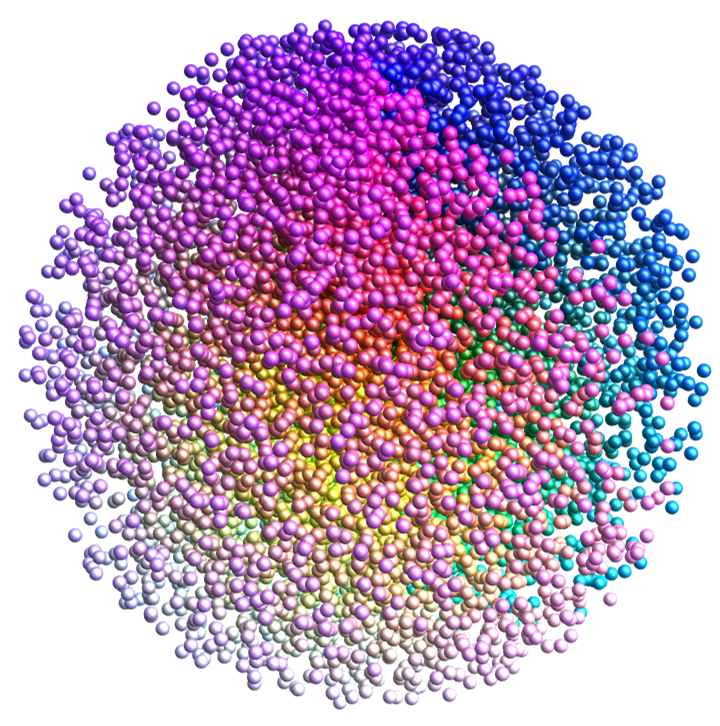Noise sphere of the legacy pseudorandom number generator used by the Wolfram Language:

 In:=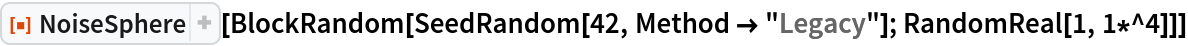Out=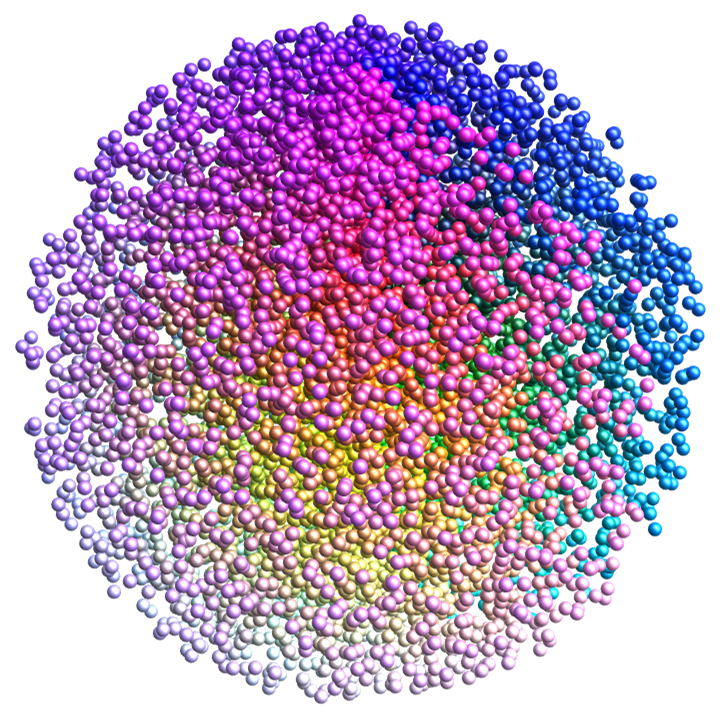The following shows an example of a poor pseudorandom sequence:

 In:=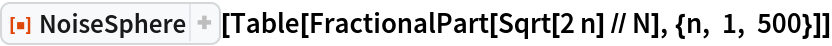Out=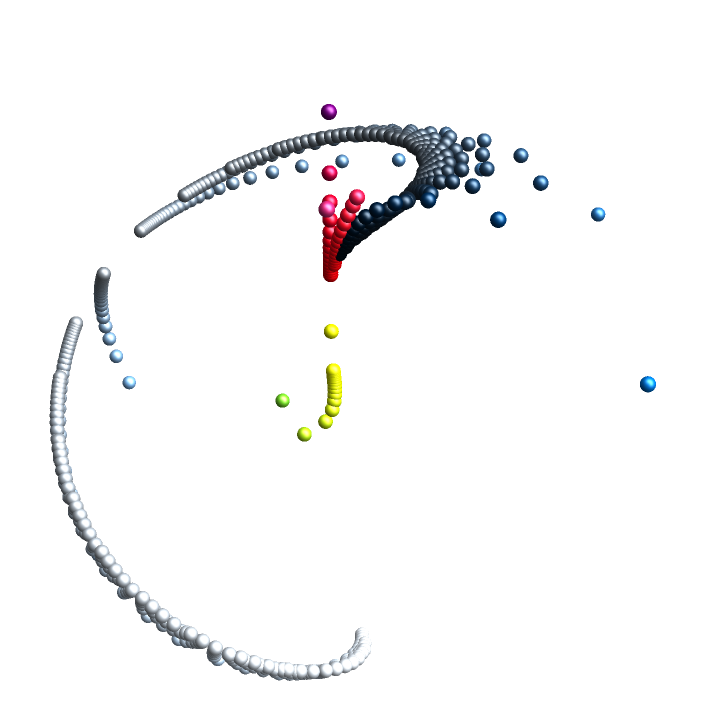## Version History

• 1.0.0 – 08 June 2021